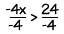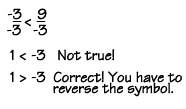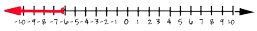Home    |    Teacher    |    Parents    |    Glossary    |    About UsSolving an inequality is very similar to solving an equation. You follow the same steps, except for one very important difference. When you multiply or divide each side of the inequality by a negative number, you have to reverse the inequality symbol! Let's try an example:

-4x > 24

Since this inequality involves multiplication, we must use the inverse, or division, to solve it. We'll divide both sides by –4 in order to leave x alone on the left side.When we simplify, because we're dividing by a negative number, we have to remember to reverse the symbol. This gives "x is less than –6," not "x is greater than –6."

x < -6

Why do we reverse the symbol? Let's see what happens if we don't. Think about the simple inequality –3 < 9. This is obviously a true statement.

-3 < 9

To demonstrate what happens when we divide by a negative number, let's divide both sides by –3. If we leave the inequality symbol the same, our answer is obviously not correct, since 1 is not less than –3.We must reverse the symbol in order to find the correct answer, which is "1 is greater than –3."

Let's go back to the original problem and graph the solution x < –6 on a number line. To graph the solution for an inequality, you start at the defining point in the inequality. Here, it's –6. Then you graph all the points that are in the solution.The red arrow shows that all the values on the number line less than –6 are in the solution. The open circle at –6 shows us that –6 is not in the solution. If the solution had been "x is less than or equal to –6," the circle would be a dark, or filled in, circle.

How can we check our answer? We can't use –6 to substitute in the inequality, because it lies outside our solution. To check, we can choose any value that lies in the solution. Let's use –7.

-4x > 24
-4(-7) > 24
28 > 24 Correct!

Our substitution gave a true result, so the solution is correct.

 Homework Help | Algebra | Equations and InequalitiesEmail this page to a friendSearch·  Solving addition and     subtraction equations·  Multiplication equations·  Division equations·  Inequalities·  Formulas·  Two-step equations     and inequalitiesFirst Glance In Depth Examples WorkoutInequalities